Math Concepts

# Can you introduce a rational number with examples?

3.3k views

 1 Introduction 2 Properties of Rational Numbers 3 Properties of Operations on Rational Numbers 4 Summary 5 About Cuemath 6 Frequently Asked Questions (FAQs) 7 External References

8 January 2021

Have you ever shared pizza with your friends? Whenever you cut it in equal parts, each part represents an equal part of the whole. This is a Rational Number.

Suppose I need to fill my new fish aquarium. The aquarium holds 50 gallons of water. How many gallons will be required to fill ⅘ of the aquarium? To find out this I need to know the rational numbers.

Calculation of interests, loans or mortgages all use rational numbers. All banking calculations need knowledge of rational numbers. The calculation of credit and debit need to be differentiated from each other. These are represented by positive and negative fractions which are rational numbers.

Depth of the submarine below sea level also needs the knowledge of negative fractions.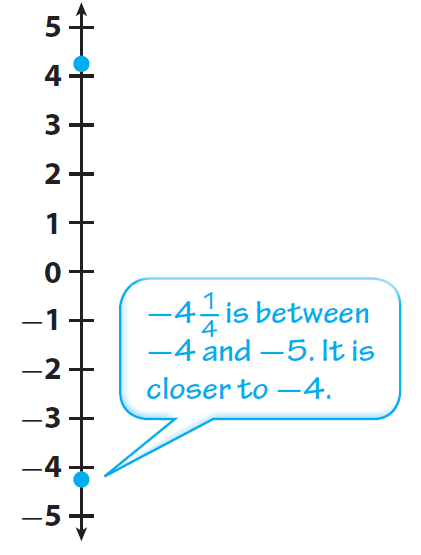In Mathematics, simple numbers which are used as counting and ordering are called Natural Numbers. Numbers 1, 2, 3, 4,……. are natural numbers. The natural numbers continue up to infinity.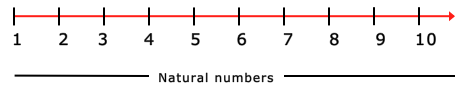Natural numbers together with zero make another set of numbers known as Whole Numbers. So 0, 1, 2, 3, 4, …….. are whole numbers. The whole numbers also go up to infinity.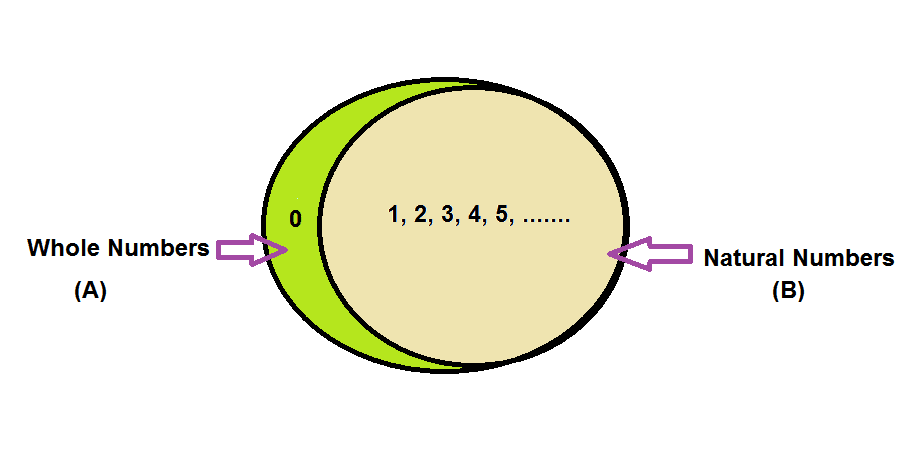All whole numbers combined with their negative counterparts (-1, -2, -3, -4, ……..) are called Integers. Integers are (……….,-4, -3, -2, -1, 0, 1, 2, 3, 4,……..). The natural numbers are called Positive Integers. Their negative counterparts are called Negative Integers. The number zero is neither positive nor negative. These numbers also can go up to infinity in both directions.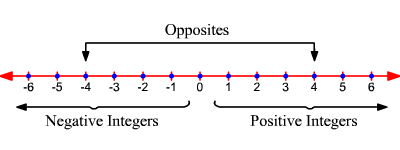The definition of numbers extends to another larger set of numbers called rational numbers. When we divide an integer with any non-zero integer that is the numbers that can be written in the form of  $$p/q$$ , where $$p$$ and $$q$$ can be integers and $$q ≠ 0$$, are called Rational Numbers.

The ratio $$p/q$$ can further be simplified and represented in decimal form.

You will see that this division of p by q gives us a terminating or a non-terminating decimal answer. This non-terminating answer will result in a repeating decimal answer. So we can define rational numbers as those decimal representations which are terminating or repeating and non-terminating numbers.

But if the decimal representation is non-repeating non-terminating then it is called an Irrational Number.

For example $$π$$: People have calculated Pi to over a quadrillion decimal places and still, there is no repeating pattern observed. The first few digits in pi look as 3.1415926535897932384626433832795.

The set of Rational and Irrational Numbers together is called Real Numbers. Download the PDF below to understand the properties of Rational Numbers.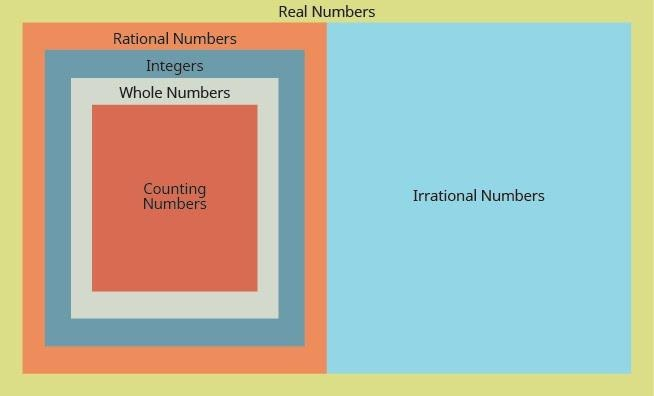Example: Which of the following options are CORRECT?
(i) Every integer is a rational number.
(ii) A rational number p/q is positive if p and q are either both positive or both negative.
(ii) A rational number p/q is negative if one of p or q is positive and the other is negative.
(iv) If there are two rational numbers with a common denominator, the one with the larger numerator is greater than the other.

(A) (i) and (iv) are incorrect

(B) (ii) and (iii) are incorrect

(C) (i) is incorrect

(D) All of them are correct

 (D) All of them are correct

## Properties of Rational Numbers

1. The word rational numbers has evolved from the word ratio. It is a ratio of two integers.

2. Rational numbers are basically the numbers that are fractions and can be represented on the number line. They can be both positive and negative.

3. All integers are also rational numbers because all the integers can be represented as $$p/q$$ with $$q =1$$.

For Example: $$7 = 7/1, -8 = -8/1$$  and likewise $$0=0/1$$, and $$0$$ is also a rational number.

4. A rational number remains unchanged when both its numerator and the denominator is multiplied/divided by a non-zero integer $$m$$.

$$pxm/qxm$$  or  $$p÷m/q÷m$$

For Example: $$2/9$$ is the same as $$2*3/9*3=6/27$$.

5. Two rational numbers can be compared in the same manner as numbers.

Example: Suppose a, b, c are rational numbers and a > b and b < c. Which of these statements is true?

(A) a > c

(B) b is the smallest rational number

(C) a < c

(D) Both (A) and (B) are correct

 (B) b is the smallest rational number

Example: Arrange the following rational numbers in ascending order: 79, 56, 1112, -13, -34.

(A) 13,  56,  1112,  79, -34

(B)  1112,  79,  56, -13, -34

(C)  -34,  -13,  79,  56,  1112

(D)   1112,  56,  79,  -13, -34

 (C)  -34,  -13,  79,  56,  1112

6. Infinite rational numbers can be calculated between any two rational numbers.

Example: The rational number that lies between 56 and 67 is _____.
(A) 12

(B) 1521

(C) 3542

(D) 7184

 (D) 7184

## Properties of Operations on Rational Numbers

### Closure Property

For any two rational numbers the results of addition, subtraction and multiplication operations give us a rational number. So we can say that the closure property holds for addition, subtraction and multiplication of rational numbers. But division by zero is not defined so closure property does not hold for the division.

### Commutative Property

If $$a= 1/2$$ and $$b= -3/4$$ then

$$a+b = b+a$$ and $$a*b = b*a$$

which can be verified by solving L.H.S. and R.H.S. separately.

$$a+b = b+a$$

$$a+b= (12) + (-34) = 24+(-34) = -14$$

$$b+a= (-34) + (12) = (-34) + 24 = -14$$

L.H.S = R.H.S

$$a*b = b*a$$

$$a*b= (12)*(-34) = -38$$

$$b*a = (-34)*(12) = -38$$

L.H.S = R.H.S

### Associative Property

The addition and Multiplication of rational numbers also follow the associative property.
$$a+(b+c)=(a+b)+c$$
$$ab*cd*ef = ab* cd* ef$$
which can be verified by solving L.H.S. and R.H.S. separately.

### Additive Inverse/ Negative of a number

For a rational number $$p/q$$, we have $$p/q + (-p/q) = (-p/q) + (p/q)=0$$
Thus we can say, $$(-p/q)$$  is the additive inverse or negative of $$( p/q)$$  and vice-versa.
This shows that the additive inverse of $$3/7$$ is $$-3/7$$  because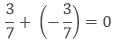### The role of 0

Zero is called the identity for the addition of rational numbers. It is called the additive identity of rational numbers.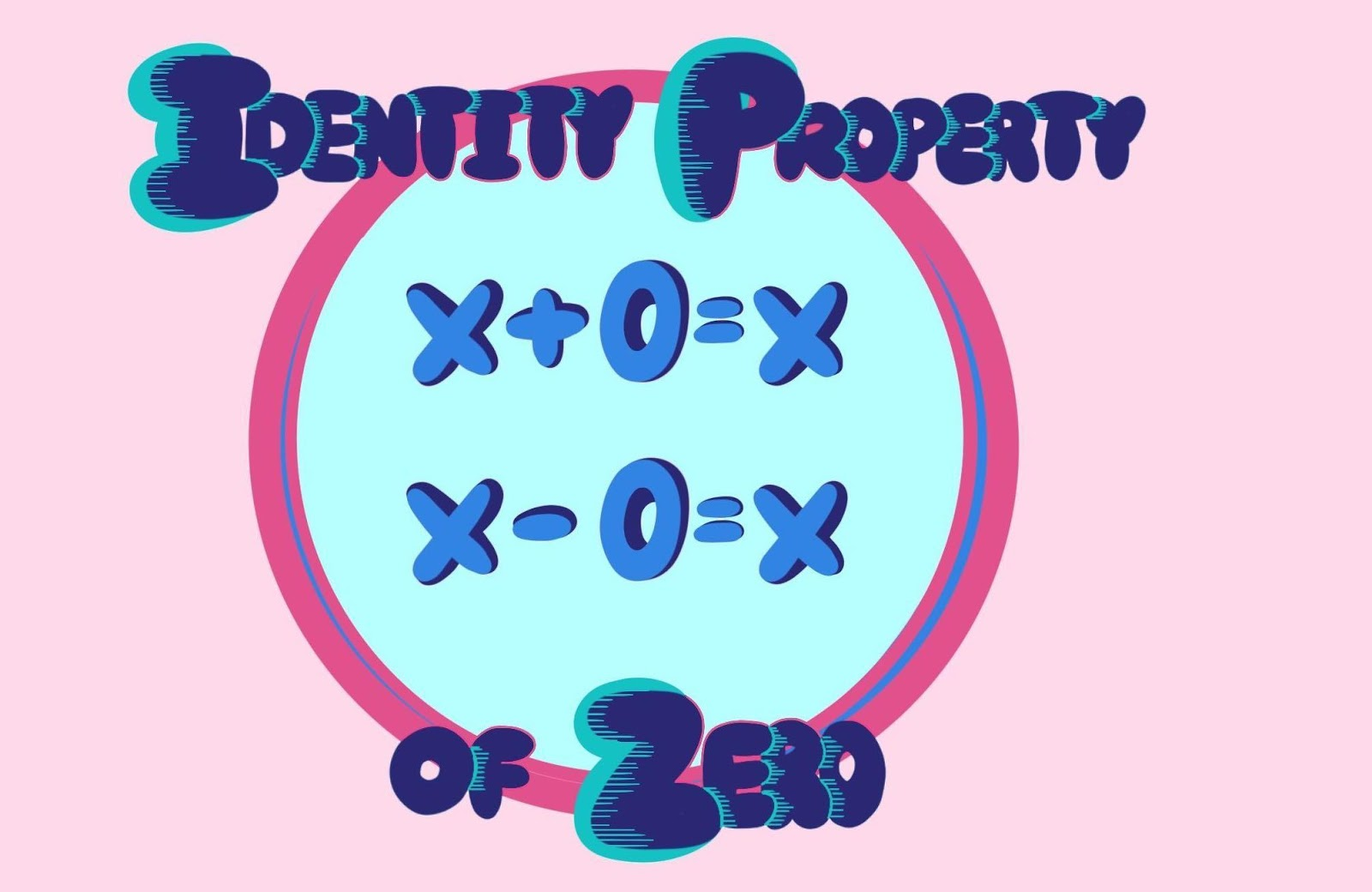### Multiplicative inverse/Reciprocal of a number

In the set of rational numbers, for every non-zero rational number $$a/b$$ there exists a rational number $$b/a$$ such that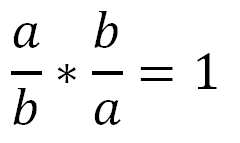so we say that $$a/b$$ has a reciprocal or multiplicative inverse given by $$b/a$$

### Distributive Property

$$a(b+c)=ab+ac$$

### The role of 1

So in rational numbers also 1 is the multiplicative identity because when we multiply any rational number with 1, we get the same number.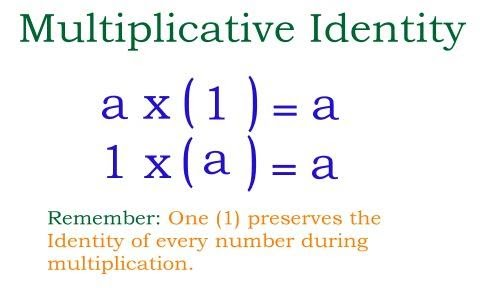## Summary

Numbers which can be written in the form of  $$p/q$$ , where $$p$$ and $$q$$ can be integers and $$q ≠ 0$$, are called Rational Numbers.

The ratio $$p/q$$ can further be simplified and represented in decimal form. They can be represented on the number line. They can be both positive and negative. The set of Rational and Irrational Numbers together is called Real Numbers.

Cuemath, a student-friendly mathematics and coding platform, conducts regular Online Live Classes for academics and skill-development, and their Mental Math App, on both iOS and Android, is a one-stop solution for kids to develop multiple skills. Understand the Cuemath Fee structure and sign up for a free trial.

## 1. Is every rational number a fraction?

No, every rational number is not a fraction unless written in the form $$p/q$$ where $$p$$ and $$q$$ can be integers and $$q ≠ 0$$.

## 2. Is zero a rational number?

Yes, zero is a rational number, if the numerator is zero (0), and the denominator is any non-zero integer, the resulting quotient is  zero.

## 3. Is $$3.14$$ a rational number?

Yes, $$3.14$$ is a rational number. It can be written in the form of $$a / b$$.

-Written by Anupama Mahajan, Cuemath Teacher